### Home > PC > Chapter 6 > Lesson 6.1.1 > Problem6-12

6-12.
1. Find the exact value of the following trig expressions. Homework Help ✎

1. csc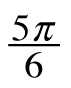2. tan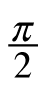3. cot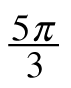4. sec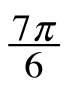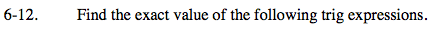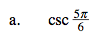1. Plot the point on a unit circle.
2. Find the sin first.
3. Flip the answer for the csc. (Find the reciprocal)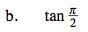$\text{Since the tan }x=\frac{\text{sin }x}{\text{cos }x},$

what issues can occur for denominator values of a fraction?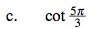Plot the point finding the side of the reference angle.
Find the tan first and take its reciprocal for the cot.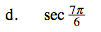Find the cos first and then its reciprocal for the sec.